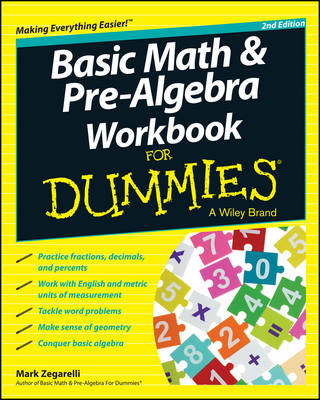Days : Hours : Minutes : Seconds•# Basic Math & Pre-algebra Workbook For Dummies® (Paperback)

(author)
£15.99
Paperback 312 Pages / Published: 04/04/2014
• Not available

This product is currently unavailable

This product is currently unavailable.

Basic Math and Pre-Algebra Workbook For Dummies, 2nd Edition helps take the guesswork out of solving math equations and will have you unraveling the mystery of FOIL in no time. Whether you need to brush up on the basics of addition, subtraction, multiplication, and division or you re ready to tackle algebraic expressions and equations, this handy workbook will demystify math so you can get back to having fun in math class. * Properly use negative numbers, units, inequalities, exponents, square roots, and absolute value * Round numbers and estimate answers * Solve problems with fractions, decimals, and percentages * Navigate basic geometry * Complete algebraic expressions and equations * Understand statistics and sets * Sample questions with step-by-step explanation * Answers to practice problems so you can check your work Let Basic Math and Pre-Algebra Workbook For Dummies, 2nd Edition take the guessing out of math and help you discover your problem solving potential.

Publisher: John Wiley & Sons Inc
ISBN: 9781118828045
Number of pages: 312
Weight: 446 g
Dimensions: 251 x 203 x 18 mm
Edition: 2nd Revised edition